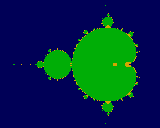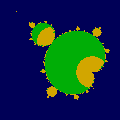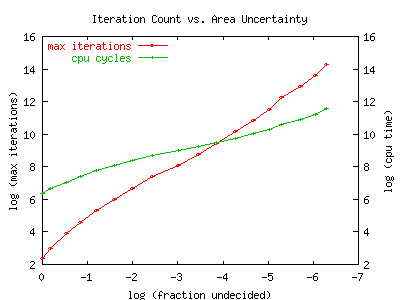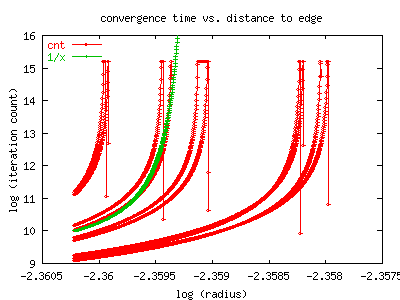# Undecidably Inside

Roger Penrose has conjectured that no algorithm exists for determining the interior of the Mandelbrot set . Stephen Smale & Lenore Blum have done work in support of this type of conjecture and the relationship of real numbers to computability ,. Simant Dube examines a special case of this conjecture for IFS . The goal of this web page is to explore a candidate for a function that unambiguously identifies the interior of the Mandelbrot set in finite time, and to try to understand its properties. The methods used here are purely experimental (i.e. computational, not theoretical). The goals are to establish:
1. Does the algorithm ever make mistakes, viz. does it ever mis-identify exterior points as belonging to the interior?
2. Does it 'eventually' identify all interior points, or are there some left unidentified?
3. Does it work in P or NP time?
4. Does it have practical applications, viz. can it be used to rapidly compute the area of the Mandelbrot set, or improve the performance of popular fractal art programs?

## The Second Derivative

The behavior of iterated points inside the Mandelbrot set is at least as interesting as the behavior of those that escape, as explored in the M-Set Derivations Room. Of particular interest is the behavior of the second derivative, shown at left:If we write the iterated Mandelbrot equation as so: Zn+1 = Zn2 + c, then, realizing that it is parameterized by c, we can take its derivative: Z'n+1 = dZn+1/dc = 2 Z'n Zn + 1. The second derivative follows: Z''n+1 = d2Zn+1/dc2 = 2 (Z''n Zn + Z'n2). Note that Zn, Z'n and Z''n are well defined for all values of c and finite n: they are entire functions, 'merely' polynomials in c. For several reasons, it is easier to work with the normalized versions of these derivatives: define Pn = Z'n / Zn and define Qn = Z''n / Zn. Note that Pn and Qn are also well defined, being ratios of polynomials, and containing at most a finite number of poles and zeros. Again, they are defined both inside and outside and on the boundary of the Mandelbrot set. This image shows the Modulus Mn of Qn: Mn = sqrt ((Re Qn)2 + (Im Qn)2). It is the dramatic difference between the interior and exterior of this image that form the basis of further exploration on this web page. (Actually, the image shows the logarithm of M4153, where red is mapped to about log(M)=21, passing through yellow, green, and down to blue-black at log(M)=-4. Taking the logarithm helps compress the color space, and bring out subtle details, especially in the interior. )

## The Interior

Of particular interest seems to the the fact that the second derivative seems to distinguish the interior from the exterior quite elegantly. But does it really, and how well? Let us iterate for N steps, and then partition the complex plane into three sets:
• The exterior: a point is marked as belonging to the exterior if it escaped (in the traditional sense) before the N'th iteration.
• The maybe-interior: a point is marked as maybe-belonging to the interior if Mn < n / (log(n)*log(n)) for some 10<n<N. (The arbitrary lower limit of 10 is imposed to avoid some low-order confusion).
• The undecided: any point not in either of the above two sets.
Some numerical tests were carried out that seem very positive. A point that is identified as 'maybe-interior' seems to never be later identified as belonging to the exterior. (This check can be made at every stage n of the iteration). The set that is 'undecided' seems to get smaller as N gets larger. The following collection of images seems to support these claims. The dark blue areas are points that have been conclusively identified as belonging to the exterior. The yellow areas are the 'undecided' points, and the dark green areas are the points that have been labelled as 'interior'. If the interior and exterior areas intersected, then they would have shown in red. Red never seems to come up.This image shows the Mandelbrot set, and stops iteration at N=210. Note the fairly wide strip of 'undecided' area separating the interior from the boundary. (Click on the image to view a larger version of the same.)Same image as above, but iterated to N=4210. Note how the 'undecided' area has narrowed dramatically.The horns tend to be a particularly troublesome area. This image helps demonstrate that the algorithm behaves well near the horns. Note that the more optimistic test Mn < n/log(n) fails near the horns (that image is not shown, but you are welcome to discover that on your own). This image also illustrates that it is quite conservative, and tends to keep a good distance from the boundary of the set. As such, it is both a relief and rather irritating: on the one hand, it seems clearly safe, and on the other, it would be far more adventurous if it were a bit more flirtatious.

Its possible that a higher derivative might converge faster; however, what we really want is something that clearly starts filling in the smaller buds visible in this picture. The fact that this computation is 'safe' near the horns seems to also prevent it from filling up small buds.Coverage of the 'baby' at (re,im)=(-1.765, 0) after 2150 iterations.Coverage of the 'baby' at (re,im)=(-0.16, 1.033) after 2150 iterations.Coverage of the 'baby' at (re,im)=(-0.16, 1.033) after 12340 iterations. Its slow going, but the area does slowly fill up.

Although more experimentation is clearly called for, this preliminary work seems to establish that this algorithm at least never mis-identifies exterior points.

## Rate of Convergence

The next question is: does the algorithm converge? That is, as the iteration count goes up, does the size of the 'undecided' area shrink at some reasonable rate?The graph seems to indicate that it does: not terribly fast, but maybe good enough. The graph is a log-log plot of the maximum iteration count vs. fraction of undecided pixels (undecided/(interior+undecided)). The fraction of undecided pixels can be thought of as an uncertainty of the area of the Mandelbrot set: its the fraction of the area which hasn't been determined to be inside or outside the set. As more and more iterations are performed, the fraction of ambiguous pixels drops. The graph appears to be nearly linear, with a slope of about 1.7. We conclude that this algorithm obtains successive digits in area of the Mandelbrot in non-polynomial time: specifically, to get M decimal digits of precision in the area, we need to iterate exp (1.7*log(10)*M) times.

The second line of the graph shows the actual number of CPU cycles needed to compute to a certain level of uncertainty. The slope appears to be about 0.8: that is, to get M decimal digits of precision in the area, we need to iterate for exp (0.8*log(10)*M) CPU cycles. The lesser slope indicates that the algorithm allows us to stop iterations early inside the set, and we need to iterate to the bitter end only near the boundary. However, the time spent iterating near the boundary quickly dominates the total CPU time: time spent deep in the interior becomes relatively small. We can conjecture that CPU time spent in some region is somehow related to some local/average fractal dimension or complexity, or vice verse might be used to define a local complexity measure.This graph shows iteration count and cpu time vs. the fraction of undecided pixels for a baby. The baby is the same as that shown pictorially, above. This is (one of ?? the??) largest baby that doesn't lie on the Re=0 axis. Note it takes considerably more cycles/iterations to start filling this in. However, the slope seems to be about the same: 1.75, which matches within measurement errors.

The slope of the cpu-usage line is about 0.9 which is similar to but slightly larger than what it was for the overall blob. I wonder if ever-smaller features become increasingly computationally challenging? Is the increase of the slope due to the challenge of the exterior, or the interior, or both?

## Convergence vs. Distance to Edge

A better sense of the rate of the convergence can be gotten by looking at the behavior on slices through the M-set. Both graphs below show ten nearly-radial slices through the 3-cycle bud located at (-0.125, 0.75).The upper graph shows how many iterations it took before a point was determined to be in the 'interior', by the measure described above. It can be clearly seen that as the edge is approached, the number of iterations needed to determined the membership of the point increases disastrously. Note that this is a log-log graph, and rather than being straight, it seems to have poles.

An alternative but undependable method of determining if a point lies inside the M-set is to attempt to detect if its orbit settled down to a cycle. This method is undependable in that there is seemingly no way to decide whether the orbit is indeed a cycle: there are exterior points which come arbitrarily close to being cycles for arbitrarily long periods of time, before diverging away. Although looking for cycles is an undependable way to identify interior points, it is interesting to compare convergence rates of this and the second-derivative method.For this bud, we measure convergence to the limit 3-cycle by counting iterations until the cycle got to within 1e-10 of itself. This figure shows that convergence to the limit cycle also becomes extremely slow as one approaches the boundary of the M-set. As the edge of the bud is approached, the number of iterations explodes far worse than exponentially, and then some. Note this is a log-log graph. Just for comparison, the green line, labelled '1/x', shows what a pole in the log would look like in comparison; that is, its a graph of exp(1/(0.0945-radius)). (The approximate radius of this bud is 0.0945, see The Bud Page for details). Although we haven't really taken the time to fit properly or correctly, the divergence looks about that bad or possibly worse.

On the basis of these graphs, it looks like the convergence of this measure is no better, and no worse, than idea of trying to detect a limit cycle. The advantage here is that the new algorithm is both a lot simpler, and seems to actually be reliable. The simplicity is clear: one does not need to guess at the period of the limit cycle one is looking for. The reliability is paramount though: exterior points that are close to the boundary will also appear to approach a limit cycle, and stay there for an arbitrarily long time, before diverging away. Without something like the second derivative, there is no way to decide if a limit cycle has been converged upon.

## Efficiency & Practical Applications

This algorithm might be useful to improve the performance of various fractal art programs ... maybe ... It is primarily useful in avoiding long iterations in the interior of the Mandelbrot set. However, it does require significant extra computational overhead (to compute the derivative) that might erase that advantage. And if the user isn't looking at something with lots of interior in the picture, then this algorithm provides no advantage at all.

This algorithm might be used to estimate the area of the Mandelbrot Set. Note that it counts the area of the babies, as well the main blob. I believe that the problem of solving for the area of the Mandelbrot set is still an 'open problem' in mathematics. It's not clear (to me) if/how this algorithm does anything to move forward on that problem.

Note that this algorithm is something like three orders of magnitude 'slower' than simply taking the area of the Mandelbrot set as everything that isn't escaped. That is, if a plain-old iteration produces 6 pixels of uncertainty, then this algorithm will still have something like 6000 pixels of uncertainty. That this is so should be quite dramatically clear from the pictures above, if you look at them & think: the M-set outline is rather crisp & sharp in all of these pictures, whereas there are still huge yellow areas of uncertainty.

## Other Approaches

Roger Bagula points out that one can track the convergence of a series to the limit cycle. If a series converges to the limit cycle, one must conclude that the point is inside the set. This, however, has problems. Not only is it computationally difficult in practice (you have to discover the limit cycle), but also doesn't work in finite time. Some exterior points will appear to approach a limit cycle, to within some arbitrary epsilon, before ultimately diverging. Picking any finite epsilon will fail to eliminate these 'false positives'. The alternative is to iterate forever, which is no alternative.

Another possibility is to compute the 'plateau'. This seems possible but difficult for the main blob. However, I am not aware of any algorithms that will also compute the baby-blobs.

## Decidability

Does this algorithm affect arguments about the decidability of the interior of the Mandelbrot set? There may indeed be undecidable points in the Mandelbrot set problem; indeed, intuitively, one senses that points 'on the boundary' are undecidable. However, the algorithm hints that the measure of the set of undecidable points is low (well less than two, near one). Hmm, lets re-examine Penrose's definition for 'non-recursive' again, and see if that's been altered ...

## Quickie Bibliography

 Roger Penrose (1989), "The Emperor's New Mind", Oxford Press.
 Blum L., Shub M. & Smale S. (1989), "On a theory of computation and complexity over the real numbers: NP completeness, recursive functions and universal machines", Bulletin of American Mathematical Society, 21, pp. 1-46.
 Simant Dube (1995), "Undecidable Problems in Fractal Geometry", http://www.csu.edu.au/ci/vol2/undecide/undecide.html
 Lenore Blum, (1990) "The Goedel Incompleteness Theorem and Decidability over a Ring"

Authored by Linas Vepstas linas@linas.org June 2000.